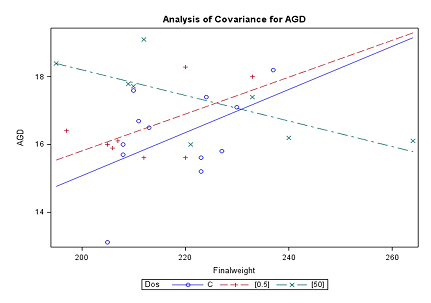13
Jan

14
Feb
Uppsala

Statistics III: Regression analysis, 4 hpThe Ph.D. course will be given in Uppsala 13 January - 14 February 2020.

Prerequisites: Statistics I or equiv.

Objective, including learning outcomes

The objective of the course is to give an overview of linear, nonlinear and nonparametric regression. On completion of the course, the student will be able to:
• specify regression models including conditions and assumptions
• select an appropriate regression model for a given problem
• carry out a regression analysis in the statistical software R or SAS
• interpret and evaluate results correctly and draw reasonable conclusions
• clearly and concisely communicate results and conclusions

Content

The course will cover the following topics:
• Simple linear regression.
• Multiple linear regression.
• Nonlinear models.
• Nonparametric regression and generalized additive models (GAM).
• Analysis of residuals.

Facts
Time: 2020-01-13 - 2020-02-14
City: Uppsala
Last signup date: 23 December 2019